Arduino

# Ultimate Beginner’s Guide to Run TFT LCD Displays by Arduino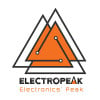December 11, 2018 by ElectroPeak Inc
Share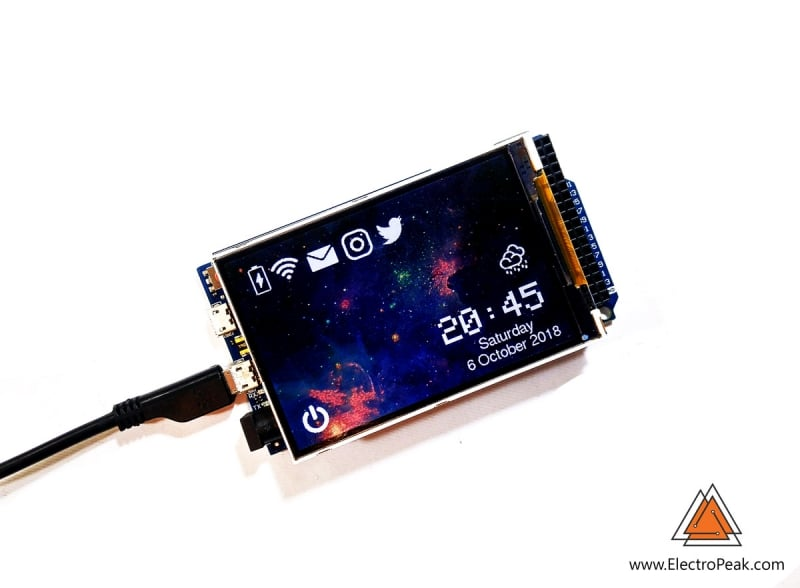Here, you will learn how to use TFT LCDs by Arduino. From basic commands to professional designs and technics are all explained here.

In this article, you will learn how to use TFT LCDs by Arduino boards. From basic commands to professional designs and technics are all explained here. At the end of this article, you can :

• Write texts and numbers with your desired font.
• Draw shapes like circle, triangle, square, etc.
• Display.bmp images on the screen.
• Change screen parameters such as rotating and inverting color.
• Display an animation by Arduino.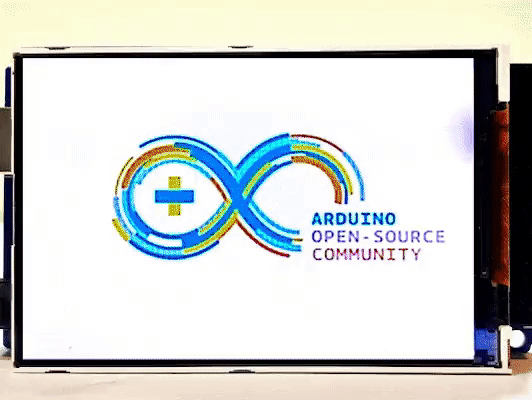## Presenting Ideas on Displays

In electronicâ€™s projects, creating an interface between user and system is very important. This interface could be created by displaying useful data, a menu, and ease of access. A beautiful design is also very important.

There are several components to achieve this. LEDs, 7-segments, Character and Graphic displays, and full-color TFT LCDs. The right component for your projects depends on the amount of data to be displayed, type of user interaction, and processor capacity.

TFT LCD is a variant of a liquid-crystal display (LCD) that uses thin-film-transistor (TFT) technology to improve image qualities such as addressability and contrast. A TFT LCD is an active matrix LCD, in contrast to passive matrix LCDs or simple, direct-driven LCDs with a few segments.

In Arduino-based projects, the processor frequency is low. So it is not possible to display complex, high definition images and high-speed motions. Therefore, full-color TFT LCDs can only be used to display simple data and commands.

In this article, we have used libraries and advanced technics to display data, charts, menu, etc. with a professional design. This can move your project presentation to a higher level.

## Which Size? Which Controller?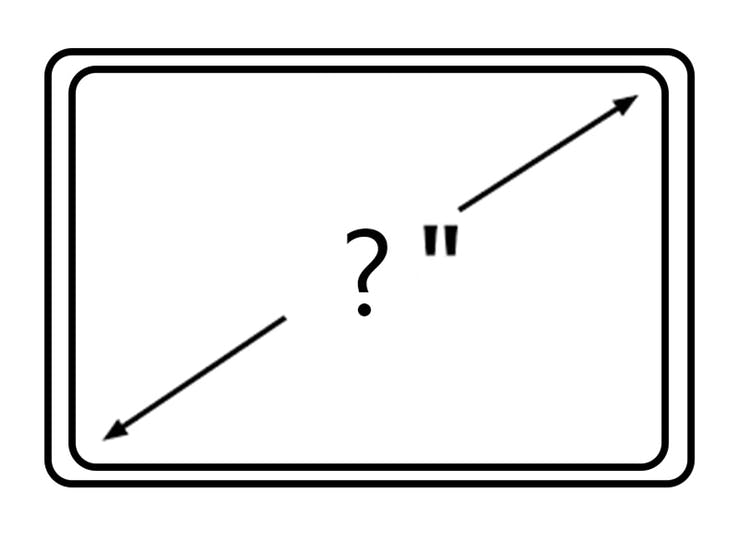Size of displays affects your project parameters. Bigger Display is not always better. if you want to display high-resolution images and signs, you should choose a big size display with higher resolution. But it decreases the speed of your processing, needs more space and also needs more current to run.

So, First, you should check the resolution, speed of motion, details of color and size of your projectâ€™s images, texts, and numbers.

We suggest popular size of Arduino displays such as 3.5 inch 480Ã—320, 2.8 inch 400Ã—240, 2.4 inch 320Ã—240 and 1.8 inch 220Ã—176.

After choosing the right display, Itâ€™s time to choose the right controller. If you want to display characters, tests, numbers and static images and the speed of display is not important, the Atmega328 Arduino boards (such as Arduino UNO) are a proper choice. If the size of your code is big, The UNO board may not be enough. You can use Arduino Mega2560 instead. And if you want to show high resolution images and motions with high speed, you should use the ARM core Arduino boards such as Arduino DUE.

## Drivers & Libraries

In electronics/computer hardware a display driver is usually a semiconductor integrated circuit (but may alternatively comprise a state machine made of discrete logic and other components) which provides an interface function between a microprocessor, microcontroller, ASIC or general-purpose peripheral interface and a particular type of display device, e.g. LCD, LED, OLED, ePaper, CRT, Vacuum fluorescent or Nixie.

The display driver will typically accept commands and data using an industry-standard general-purpose serial or parallel interface, such as TTL, CMOS, RS232, SPI, I2C, etc. and generate signals with suitable voltage, current, timing and demultiplexing to make the display show the desired text or image.

Unzip the MCUFRIEND KBV and open the MCUFRIEND_kbv.CPP. You can see the list of drivers that are supported by MCUFRIEND library.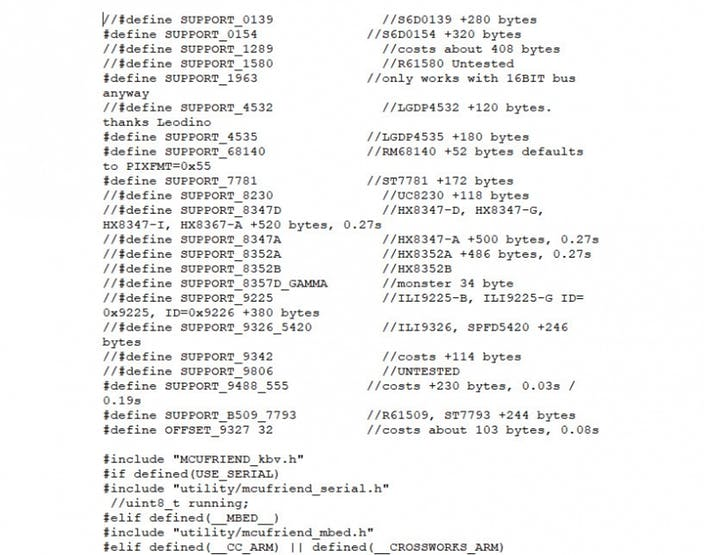Open Example folder. There are several example codes that you can run by Arduino. Hook up the LCD and test some of the examples.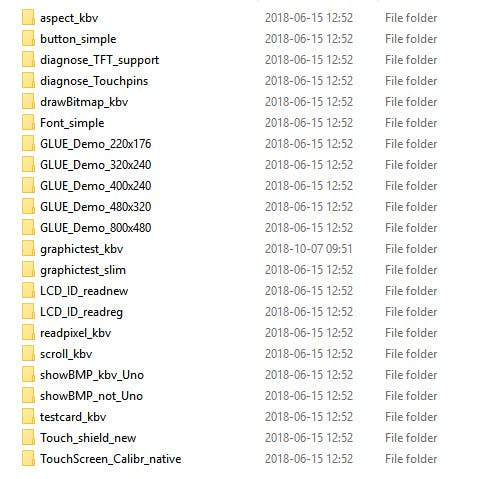## Code

You must add the library and then upload the code. If it is the first time you run an Arduino board, donâ€™t worry. Just follow these steps:

• Go to www.arduino.cc/en/Main/Software and download the software of your OS. Install the IDE software as instructed.
• Run the Arduino IDE and clear the text editor and copy the following code in the text editor.
• Navigate to sketch and include the libraries. Now click add ZIP library and add the libraries
• Choose the board in tools and boards, select your Arduino Board.
• Connect the Arduino to your PC and set the COM port in tools and port.
• Press the Upload (Arrow sign) button.
• You are all set!

After uploading an example code, itâ€™s time to learn how to create your images on the LCD.

Open a new Sketch, and the necessary codes as described in the following sections.

## Library

``````#include "Adafruit_GFX.h"
#include "MCUFRIEND_kbv.h"``````

The first line adds core graphics library for displays (written by Adafruit).

The second adds a library that supports drivers of MCUFRIEND Arduino display shields.

``````#include "TouchScreen.h" // only when you want to use touch screen
#include "bitmap_mono.h" // when you want to display a bitmap image from library
#include "bitmap_RGB.h" // when you want to display a bitmap image from library
#include "Fonts/FreeSans9pt7b.h"    // when you want other fonts
#include "Fonts/FreeSans12pt7b.h" // when you want other fonts
#include "Fonts/FreeSerif12pt7b.h" // when you want other fonts
#include "FreeDefaultFonts.h" // when you want other fonts
#include "SPI.h"  // using sdcard for display bitmap image
#include "SD.h"``````

These libraries are not necessary for now, but you can add them.

## Basic Commands

### Class & Object

``````//(int CS=A3, int RS=A2, int WR=A1, int RD=A0, int RST=A4)
MCUFRIEND_kbv tft(A3, A2, A1, A0, A4);``````

This line makes an object named TFT from MCUFRIEND_kbv class and provides an SPI communication between LCD and Arduino.

### Running the LCD

``````uint16_t ID = tft.readID();
tft.begin(ID);``````

The tft.readID function reads ID from the display and put it in ID variable. Then tft.begin function gets ID and the LCD gets ready to work.

### Resolution of the Display

``````tft.width(); //int16_t width(void);
tft.height(); //int16_t height(void);``````

By these two functions, You can find out the resolution of the display. Just add them to the code and put the outputs in a uint16_t variable. Then read it from the Serial port by Serial.println();. First add Serial.begin(9600); in setup().

### Color of the Screen

``tft.fillScreen(t); //fillScreen(uint16_t t);``

fillScreen function change the color of screen to t color. The t should be a 16bit variable containing UTFT color code.

``````#define BLACK 0x0000
#define NAVY 0x000F
#define DARKGREEN 0x03E0
#define DARKCYAN 0x03EF
#define MAROON 0x7800
#define PURPLE 0x780F
#define OLIVE 0x7BE0
#define LIGHTGREY 0xC618
#define DARKGREY 0x7BEF
#define BLUE 0x001F
#define GREEN 0x07E0
#define CYAN 0x07FF
#define RED 0xF800
#define MAGENTA 0xF81F
#define YELLOW 0xFFE0
#define WHITE 0xFFFF
#define ORANGE 0xFD20
#define GREENYELLOW 0xAFE5
#define PINK 0xF81F``````

You can add these lines to the top of your code and just use the name of the color in the functions.

### Filling Pixels

``````tft.drawPixel(x,y,t); //drawPixel(int16_t x, int16_t y, uint16_t t)

drawPixel function fills a pixel in x and y location by t color.

readPixel function read the color of a pixel in x and y location.

### Drawing Lines

``````tft.drawFastVLine(x,y,h,t);
//drawFastVLine(int16_t x, int16_t y, int16_t h, uint16_t t)
tft.drawFastHLine(x,y,w,t);
//drawFastHLine(int16_t x, int16_t y, int16_t w, uint16_t t)
tft.drawLine(xi,yi,xj,yj,t);
//drawLine(int16_t x0, int16_t y0, int16_t x1, int16_t y1, uint16_t t)``````

drawFastVLine function draws a vertical line that starts in x, y location, and its length is h pixel and its color is t.

drawFastHLine function draws a horizontal line that starts in x and y location and the length is w pixel and the color is t.

drawLine function draws a line that starts in xi and yi locationends is in xj and yj and the color is t.

``````for (uint16_t a=0; a<5; a++)
{    tft.drawFastVLine(x+a, y, h, t);}
for (uint16_t a=0; a<5; a++)
{    tft.drawFastHLine(x, y+a, w, t);}
for (uint16_t a=0; a<5; a++)
{    tft.drawLine(xi+a, yi, xj+a, yj, t);}
for (uint16_t a=0; a<5; a++)
{    tft.drawLine(xi, yi+a, xj, yj+a, t);}``````

These three blocks of code draw lines like the previous code with 5-pixel thickness.

``````tft.fillRect(x,y,w,h,t);
//fillRect(int16_t x, int16_t y, int16_t w, int16_t h, uint16_t t)
tft.drawRect(x,y,w,h,t);
//drawRect(int16_t x, int16_t y, int16_t w, int16_t h, uint16_t t)
tft.fillRoundRect(x,y,w,h,r,t);
//fillRoundRect (int16_t x, int16_t y, int16_t w, int16_t h, uint8_t R , uint16_t t)
tft.drawRoundRect(x,y,w,h,r,t);
//drawRoundRect(int16_t x, int16_t y, int16_t w, int16_t h, uint8_t R , uint16_t t)``````

fillRect function draws a filled rectangle in x and y location. w is width, h is height and t is color of the rextangle

drawRect function draws a rectangle in x and y location with w width and h height and t color.

fillRoundRect function draws a filled Rectangle with r radius round corners in x and y location and w width and h height and t color.

drawRoundRect function draws a Rectangle with r radius round corners in x and y location and w width and h height and t color.

### Drawing Circles

``````tft.drawCircle(x,y,r,t); //drawCircle(int16_t x, int16_t y, int16_t r, uint16_t t)
tft.fillCircle(x,y,r,t); //fillCircle(int16_t x, int16_t y, int16_t r, uint16_t t)``````

drawCircle function draws a circle in x and y location and r radius and t color.

fillCircle function draws a filled circle in x and y location and r radius and t color.

``````for (int p = 0; p < 4000; p++)
{  j = 120 * (sin(PI * p / 2000));
i = 120 * (cos(PI * p / 2000));
j2 = 60 * (sin(PI * p / 2000));
i2 = 60 * (cos(PI * p / 2000));
tft.drawLine(i2 + 160, j2 + 160, i + 160, j + 160, col[n]);
}``````

By this code, you can draw an Arc. change the â€œforâ€ between 0 and 4000.

### Drawing Triangles

``````tft.drawTriangle(x1,y1,x2,y2,x3,y3,t);
//drawTriangle(int16_t x1, int16_t y1, int16_t x2, int16_t y2, int16_t x3, int16_t y3,// uint16_t t)
tft.fillTriangle(x1,y1,x2,y2,x3,y3,t);
//fillTriangle(int16_t x1, int16_t y1, int16_t x2, int16_t y2, int16_t x3, int16_t y3,// uint16_t t)``````

drawTriangle function draws a triangle with three corner location x, y and z, and t color.

fillTriangle function draws a filled triangle with three corner location x, y and z, and t color.

### Displaying Text

``tft.setCursor(x,y); //setCursor(int16_t x, int16_t y)``

This code sets the cursor position to of x and y

``````tft.setTextColor(t); //setTextColor(uint16_t t)
tft.setTextColor(t,b); //setTextColor(uint16_t t, uint16_t b)``````

The first line sets the color of the text. Next line sets the color of text and its background.

``tft.setTextSize(s); //setTextSize(uint8_t s)``

This code sets the size of text by s. s is a number between 1 and 5.

``tft.write(c); //write(uint8_t c)``

This code displays a character.

``````tft.println("www.Electropeak.com");
tft.print("www.Electropeak.com");``````

The first function displays a string and moves the cursor to the next line.

The second function just displays the string.

``````showmsgXY(x,y,sz,&FreeSans9pt7b,"www.Electropeak.com");
//void showmsgXY(int x, int y, int sz, const GFXfont *f, const char *msg)
void showmsgXY(int x, int y, int sz, const GFXfont *f, const char *msg)
{  uint16_t x1, y1;
uint16_t wid, ht;
tft.setFont(f);
tft.setCursor(x, y);
tft.setTextColor(0x0000);
tft.setTextSize(sz);
tft.print(msg);
}``````

This function changes the font of the text. You should add this function and font libraries.

``````for (int j = 0; j < 20; j++) {
tft.setCursor(145, 290);
int color = tft.color565(r -= 12, g -= 12, b -= 12);
tft.setTextColor(color);
tft.print("www.Electropeak.com");
delay(30);
}``````

### Rotating the Screen

``tft.setRotation(r); //setRotation(uint8_t r)``

This code rotates the screen. 0=0, 1=90, 2=180, 3=270.

### Inverting Screen Colors

``tft.invertDisplay(i); //invertDisplay(boolean i)``

This code inverts the colors of the screen.

``tft.color565(r,g,b); //uint16_t color565(uint8_t r, uint8_t g, uint8_t b)``

This code give RGB code and get UTFT color code.

### Scrolling the Screen

``````for (uint16_t i = 0; i < maxscroll; i++) {
tft.vertScroll(0, maxscroll, i);
delay(10);}``````

This code Scroll your screen. The Maxroll is the maximum height of your scrolling.

### Reset

``tft.reset();``

This code resets the screen.

### Displaying Monochrome Images

``````static const uint8_t name[] PROGMEM =
}
tft.drawBitmap(x, y, name, sx, sy, 0x0000);``````

First you should convert your image to hex code. Download the software from the following link. if you donâ€™t want to change the settings of the software, you must invert the color of the image and make the image horizontally mirrored and rotate it 90 degrees counterclockwise. Now add it to the software and convert it. Open the exported file and copy the hex code to Arduino IDE. x and y are locations of the image. sx and sy are sizes of image. you can change the color of the image in the last input.

### RGB Color Image Displaying

``````const uint16_t name[] PROGMEM = {
}
tft.drawRGBBitmap(x, y, name, sx, sy);``````

First you should convert your image to code.Use this link to convert the image:

http://www.rinkydinkelectronics.com/t_imageconverter565.php

Upload your image and download the converted file that the UTFT libraries can process. Now copy the hex code to Arduino IDE. x and y are locations of the image. sx and sy are size of the image.

## Predesigned Templates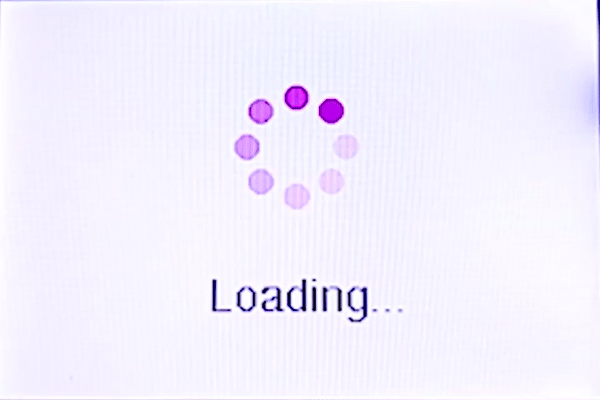In this template, We just used a string and 8 filled circles that change their colors in order. To draw circles around a static point, You can use sin(); and cos(); functions. you should define the PI number. To change colors, you can use color565(); function and replace your RGB code.

``````#include "Adafruit_GFX.h"
#include "MCUFRIEND_kbv.h"
MCUFRIEND_kbv tft;
#include "Fonts/FreeSans9pt7b.h"
#include "Fonts/FreeSans12pt7b.h"
#include "Fonts/FreeSerif12pt7b.h"
#include "FreeDefaultFonts.h"
#define PI 3.1415926535897932384626433832795
int col;
void showmsgXY(int x, int y, int sz, const GFXfont *f, const char *msg)
{
int16_t x1, y1;
uint16_t wid, ht;
tft.setFont(f);
tft.setCursor(x, y);
tft.setTextColor(0x0000);
tft.setTextSize(sz);
tft.print(msg);
}
void setup() {
tft.reset();
Serial.begin(9600);
tft.begin(ID);
tft.setRotation(1);
tft.invertDisplay(true);
tft.fillScreen(0xffff);
col = tft.color565(155, 0, 50);
col = tft.color565(170, 30, 80);
col = tft.color565(195, 60, 110);
col = tft.color565(215, 90, 140);
col = tft.color565(230, 120, 170);
col = tft.color565(250, 150, 200);
col = tft.color565(255, 180, 220);
col = tft.color565(255, 210, 240);
}
void loop() {
for (int i = 8; i > 0; i--) {
tft.fillCircle(240 + 40 * (cos(-i * PI / 4)), 120 + 40 * (sin(-i * PI / 4)), 10, col); delay(15);
tft.fillCircle(240 + 40 * (cos(-(i + 1)*PI / 4)), 120 + 40 * (sin(-(i + 1)*PI / 4)), 10, col); delay(15);
tft.fillCircle(240 + 40 * (cos(-(i + 2)*PI / 4)), 120 + 40 * (sin(-(i + 2)*PI / 4)), 10, col); delay(15);
tft.fillCircle(240 + 40 * (cos(-(i + 3)*PI / 4)), 120 + 40 * (sin(-(i + 3)*PI / 4)), 10, col); delay(15);
tft.fillCircle(240 + 40 * (cos(-(i + 4)*PI / 4)), 120 + 40 * (sin(-(i + 4)*PI / 4)), 10, col); delay(15);
tft.fillCircle(240 + 40 * (cos(-(i + 5)*PI / 4)), 120 + 40 * (sin(-(i + 5)*PI / 4)), 10, col); delay(15);
tft.fillCircle(240 + 40 * (cos(-(i + 6)*PI / 4)), 120 + 40 * (sin(-(i + 6)*PI / 4)), 10, col); delay(15);
tft.fillCircle(240 + 40 * (cos(-(i + 7)*PI / 4)), 120 + 40 * (sin(-(i + 7)*PI / 4)), 10, col); delay(15);
}
}``````

#### Logo Presentation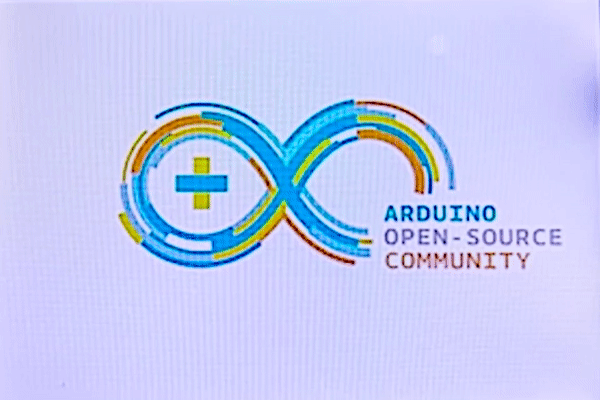In this template, We converted a.jpg image to.c file and added to the code, wrote a string and used the fade code to display. Then we used scroll code to move the screen left. Download the.h file and add it to the folder of the Arduino sketch.

``````#include "Adafruit_GFX.h"    // Core graphics library
#include "MCUFRIEND_kbv.h"   // Hardware-specific library
MCUFRIEND_kbv tft;
#include "Ard_Logo.h"
#define BLACK   0x0000
#define RED     0xF800
#define GREEN   0x07E0
#define WHITE   0xFFFF
#define GREY    0x8410
#include "Fonts/FreeSans9pt7b.h"
#include "Fonts/FreeSans12pt7b.h"
#include "Fonts/FreeSerif12pt7b.h"
#include "FreeDefaultFonts.h"
void showmsgXY(int x, int y, int sz, const GFXfont *f, const char *msg)
{
int16_t x1, y1;
uint16_t wid, ht;
tft.setFont(f);
tft.setCursor(x, y);
tft.setTextSize(sz);
tft.println(msg);
}
uint8_t r = 255, g = 255, b = 255;
uint16_t color;
void setup()
{
Serial.begin(9600);
tft.begin(ID);
tft.invertDisplay(true);
tft.setRotation(1);
}
void loop(void)
{
tft.invertDisplay(true);
tft.fillScreen(WHITE);
tft.drawRGBBitmap(100, 50, Logo, 350, 200);
delay(1000);
tft.setTextSize(2);
for (int j = 0; j < 20; j++) {
color = tft.color565(r -= 12, g -= 12, b -= 12);
tft.setTextColor(color);
showmsgXY(95, 280, 1, &FreeSans12pt7b, "ELECTROPEAK PRESENTS");
delay(20);
}
delay(1000);
for (int i = 0; i < 480; i++) {
tft.vertScroll(0, 480, i);
tft.drawFastVLine(i, 0, 320, 0xffff); // vertical line
delay(5);}
while (1);
}``````

Dot Chart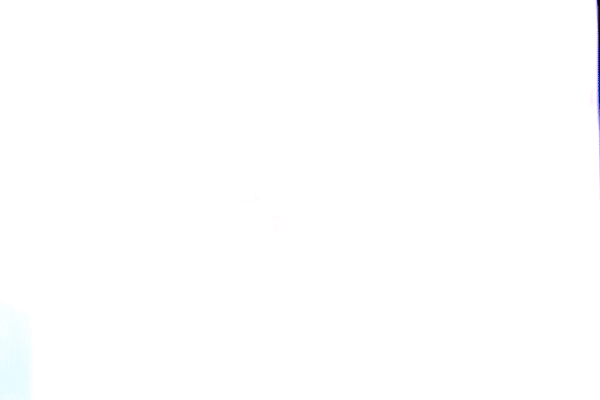In this template, We used draw lines, filled circles, and string display functions.

``````#include "Adafruit_GFX.h"
#include "MCUFRIEND_kbv.h"
MCUFRIEND_kbv tft;
uint16_t ox=0,oy=0;
int ave=0, avec=0, avet=0;
////////////////////////////////////////////////////////////////
void aveg(void)
{int z=0;
Serial.println(ave);
Serial.println(avec);
avet=ave/avec;
Serial.println(avet);
avet=avet*32;
for (int i=0; i<24; i++){
for (uint16_t a=0; a<3; a++){
tft.drawLine(avet+a, z, avet+a, z+10, 0xFB21);} // thick
for (uint16_t a=0; a<2; a++){ tft.drawLine(avet-a, z, avet-a, z+10, 0xFB21);} delay(100); z=z+20; } } ////////////////////////////////////////////////////////////////// void dchart_10x10(uint16_t nx,uint16_t ny) { ave+=nx; avec++; nx=nx*32; ny=ny*48; tft.drawCircle(nx, ny, 10, 0x0517); tft.drawCircle(nx, ny, 9, 0x0517); tft.fillCircle(nx, ny, 7, 0x0517); delay (100); ox=nx; oy=ny; } /////////////////////////////////////////////////////////////////////// void dotchart_10x10(uint16_t nx,uint16_t ny) { ave+=nx; avec++; nx=nx*32; ny=ny*48; int plus=0; float fplus=0; int sign=0; int y=0,x=0; y=oy; x=ox; float xmines, ymines; xmines=nx-ox; ymines=ny-oy; if (ox>nx)
{xmines=ox-nx;
sign=1;}
else
sign=0;
for (int a=0; a<(ny-oy); a++)
{
fplus+=xmines/ymines;
plus=fplus;
if (sign==1)
tft.drawFastHLine(0, y, x-plus, 0xBFDF);
else
tft.drawFastHLine(0, y, x+plus, 0xBFDF);
y++;
delay(5);}
for (uint16_t a=0; a<2; a++){
tft.drawLine(ox+a, oy, nx+a, ny, 0x01E8);} // thick
for (uint16_t a=0; a<2; a++){
tft.drawLine(ox, oy+a, nx, ny+a, 0x01E8);}
ox=nx;
oy=ny;
}
////////////////////////////////////////////////////////////////////
void setup() {
tft.reset();
Serial.begin(9600);
tft.begin(ID);
}
void loop() {
tft.invertDisplay(true);
tft.fillScreen(0xffff);
dotchart_10x10(3, 0);
dotchart_10x10(2, 1);
dotchart_10x10(4, 2);
dotchart_10x10(4, 3);
dotchart_10x10(5, 4);
dotchart_10x10(3, 5);
dotchart_10x10(6, 6);
dotchart_10x10(7, 7);
dotchart_10x10(9, 8);
dotchart_10x10(8, 9);
dotchart_10x10(10, 10);
dchart_10x10(3, 0);
dchart_10x10(2, 1);
dchart_10x10(4, 2);
dchart_10x10(4, 3);
dchart_10x10(5, 4);
dchart_10x10(3, 5);
dchart_10x10(6, 6);
dchart_10x10(7, 7);
dchart_10x10(9, 8);
dchart_10x10(8, 9);
dchart_10x10(10, 10);
tft.setRotation(1);
tft.setTextSize(2);
tft.setTextColor(0x01E8);
tft.setCursor(20, 20);
tft.print("Average");
int dl=20;
for (int i=0;i<6;i++){
for (uint16_t a=0; a<3; a++){
tft.drawLine(dl, 40+a, dl+10, 40+a, 0xFB21);}
dl+=16;}
tft.setRotation(0);
aveg();
while(1);
}``````

You can find more chart templates here.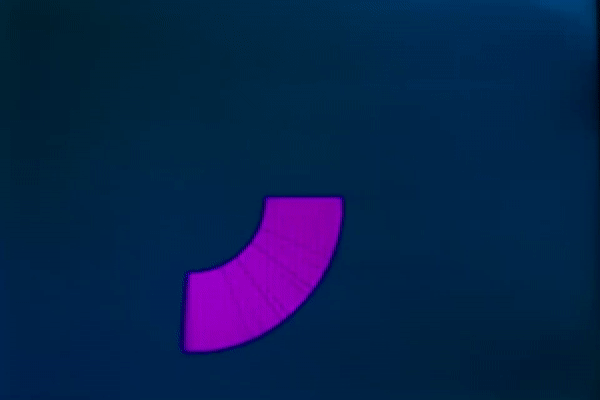Music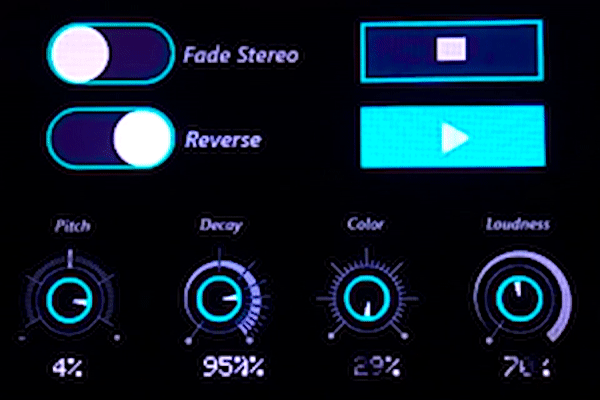In this template, We added a converted image to code and then used two black and white arcs to create the pointer of volumes. Download the.h file and add it to the folder of the Arduino sketch.

``````#include "Adafruit_GFX.h"
#include "MCUFRIEND_kbv.h"
MCUFRIEND_kbv tft;
#include "Volume.h"
#define BLACK   0x0000
int a = 0,b = 4000,c = 1000,d = 3000;
int s=2000;
int j, j2;
int i, i2;
int White;
void setup()
{
Serial.begin(9600);
tft.begin(ID);
tft.invertDisplay(true);
tft.setRotation(1);
}
void loop(void)
{
tft.invertDisplay(true);
tft.fillScreen(BLACK);
tft.drawRGBBitmap(0, 0, test, 480, 320);
White = tft.color565(255, 255, 255);
while(1){
if (a < s) {
j = 14 * (sin(PI * a / 2000));
i = 14 * (cos(PI * a / 2000));
j2 = 1 * (sin(PI * a / 2000));
i2 = 1 * (cos(PI * a / 2000));
tft.drawLine(i2 + 62, j2 + 240, i + 62, j + 240, White);
j = 14 * (sin(PI * (a-300) / 2000));
i = 14 * (cos(PI * (a-300) / 2000));
j2 = 1 * (sin(PI * (a-300) / 2000));
i2 = 1 * (cos(PI * (a-300) / 2000));
tft.drawLine(i2 + 62, j2 + 240, i + 62, j + 240, 0x0000);
tft.fillRect(50, 285, 30, 30, 0x0000);
tft.setTextSize(2);
tft.setTextColor(0xffff);
tft.setCursor(50, 285);
tft.print(a / 40); tft.print("%");
a++;
}
if (b < s) {
j = 14 * (sin(PI * b / 2000));
i = 14 * (cos(PI * b / 2000));
j2 = 1 * (sin(PI * b / 2000));
i2 = 1 * (cos(PI * b / 2000));
tft.drawLine(i2 + 180, j2 + 240, i + 180, j + 240, White);
j = 14 * (sin(PI * (b-300) / 2000));
i = 14 * (cos(PI * (b-300) / 2000));
j2 = 1 * (sin(PI * (b-300) / 2000));
i2 = 1 * (cos(PI * (b-300) / 2000));
tft.drawLine(i2 + 180, j2 + 240, i + 180, j + 240, 0x0000);
tft.fillRect(168, 285, 30, 30, 0x0000);
tft.setTextSize(2);
tft.setTextColor(0xffff);
tft.setCursor(168, 285);
tft.print(b / 40); tft.print("%");
b++;}
if (c < s) {
j = 14 * (sin(PI * c / 2000));
i = 14 * (cos(PI * c / 2000));
j2 = 1 * (sin(PI * c / 2000));
i2 = 1 * (cos(PI * c / 2000));
tft.drawLine(i2 + 297, j2 + 240, i + 297, j + 240, White);
j = 14 * (sin(PI * (c-300) / 2000));
i = 14 * (cos(PI * (c-300) / 2000));
j2 = 1 * (sin(PI * (c-300) / 2000));
i2 = 1 * (cos(PI * (c-300) / 2000));
tft.drawLine(i2 + 297, j2 + 240, i + 297, j + 240, 0x0000);
tft.fillRect(286, 285, 30, 30, 0x0000);
tft.setTextSize(2);
tft.setTextColor(0xffff);
tft.setCursor(286, 285);
tft.print(c / 40); tft.print("%");
c++;}
if (d < s) { j = 14 * (sin(PI * d / 2000)); i = 14 * (cos(PI * d / 2000)); j2 = 1 * (sin(PI * d / 2000)); i2 = 1 * (cos(PI * d / 2000)); tft.drawLine(i2 + 414, j2 + 240, i + 414, j + 240, White); j = 14 * (sin(PI * (d-300) / 2000)); i = 14 * (cos(PI * (d-300) / 2000)); j2 = 1 * (sin(PI * (d-300) / 2000)); i2 = 1 * (cos(PI * (d-300) / 2000)); tft.drawLine(i2 + 414, j2 + 240, i + 414, j + 240, 0x0000); tft.fillRect(402, 285, 30, 30, 0x0000); tft.setTextSize(2); tft.setTextColor(0xffff); tft.setCursor(402, 285); tft.print(d / 40); tft.print("%"); d++;} if (a > s) {
j = 14 * (sin(PI * a / 2000));
i = 14 * (cos(PI * a / 2000));
j2 = 1 * (sin(PI * a / 2000));
i2 = 1 * (cos(PI * a / 2000));
tft.drawLine(i2 + 62, j2 + 240, i + 62, j + 240, White);
j = 14 * (sin(PI * (a+300) / 2000));
i = 14 * (cos(PI * (a+300) / 2000));
j2 = 1 * (sin(PI * (a+300) / 2000));
i2 = 1 * (cos(PI * (a+300) / 2000));
tft.drawLine(i2 + 62, j2 + 240, i + 62, j + 240, 0x0000);
tft.fillRect(50, 285, 30, 30, 0x0000);
tft.setTextSize(2);
tft.setTextColor(0xffff);
tft.setCursor(50, 285);
tft.print(a / 40); tft.print("%");
a--;}
if (b > s) {
j = 14 * (sin(PI * b / 2000));
i = 14 * (cos(PI * b / 2000));
j2 = 1 * (sin(PI * b / 2000));
i2 = 1 * (cos(PI * b / 2000));
tft.drawLine(i2 + 180, j2 + 240, i + 180, j + 240, White);
j = 14 * (sin(PI * (b+300) / 2000));
i = 14 * (cos(PI * (b+300) / 2000));
j2 = 1 * (sin(PI * (b+300) / 2000));
i2 = 1 * (cos(PI * (b+300) / 2000));
tft.drawLine(i2 + 180, j2 + 240, i + 180, j + 240, 0x0000);
tft.fillRect(168, 285, 30, 30, 0x0000);
tft.setTextSize(2);
tft.setTextColor(0xffff);
tft.setCursor(168, 285);
tft.print(b / 40); tft.print("%");
b--;}
if (c > s) {
j = 14 * (sin(PI * c / 2000));
i = 14 * (cos(PI * c / 2000));
j2 = 1 * (sin(PI * c / 2000));
i2 = 1 * (cos(PI * c / 2000));
tft.drawLine(i2 + 297, j2 + 240, i + 297, j + 240, White);
j = 14 * (sin(PI * (c+300) / 2000));
i = 14 * (cos(PI * (c+300) / 2000));
j2 = 1 * (sin(PI * (c+300) / 2000));
i2 = 1 * (cos(PI * (c+300) / 2000));
tft.drawLine(i2 + 297, j2 + 240, i + 297, j + 240, 0x0000);
tft.fillRect(286, 285, 30, 30, 0x0000);
tft.setTextSize(2);
tft.setTextColor(0xffff);
tft.setCursor(286, 285);
tft.print(c / 40); tft.print("%");
c--;}
if (d > s) {
j = 14 * (sin(PI * d / 2000));
i = 14 * (cos(PI * d / 2000));
j2 = 1 * (sin(PI * d / 2000));
i2 = 1 * (cos(PI * d / 2000));
tft.drawLine(i2 + 414, j2 + 240, i + 414, j + 240, White);
j = 14 * (sin(PI * (d+300) / 2000));
i = 14 * (cos(PI * (d+300) / 2000));
j2 = 1 * (sin(PI * (d+300) / 2000));
i2 = 1 * (cos(PI * (d+300) / 2000));
tft.drawLine(i2 + 414, j2 + 240, i + 414, j + 240, 0x0000);
tft.fillRect(402, 285, 30, 30, 0x0000);
tft.setTextSize(2);
tft.setTextColor(0xffff);
tft.setCursor(402, 285);
tft.print(d / 40); tft.print("%");
d--;}
}
}``````

You can find more animated templates (Like the following GIFs) by clicking on this link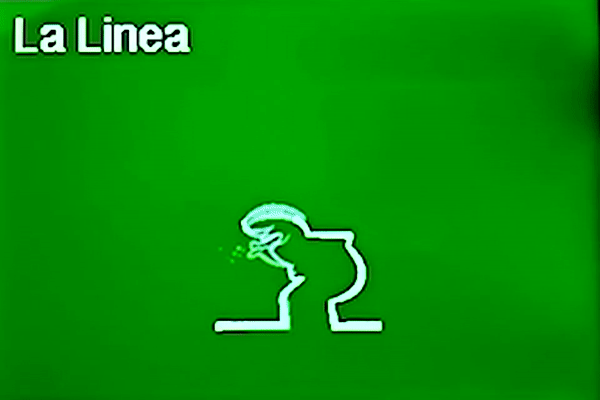#### Screen Saver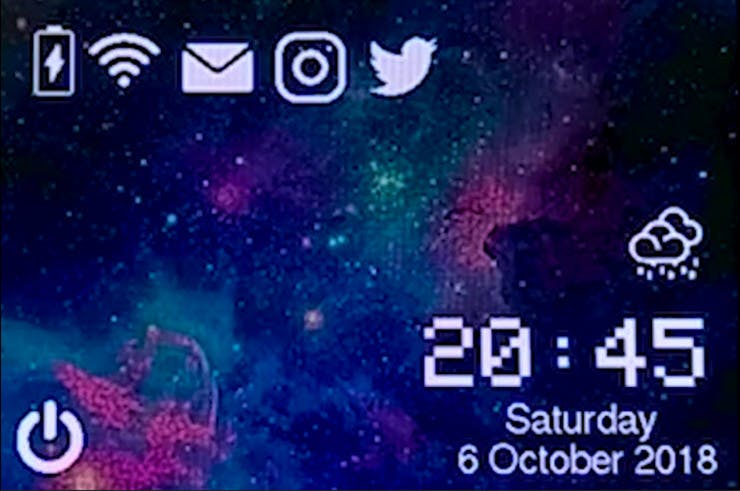In this template, We just display some images by RGBbitmap and bitmap functions. Just make a code for touchscreen and use this template. Download the.h file and add it to folder of the Arduino sketch.

``````#include "Adafruit_GFX.h"    // Core graphics library
#include "MCUFRIEND_kbv.h"   // Hardware-specific library
MCUFRIEND_kbv tft;
#define BLACK   0x0000
#define RED     0xF800
#define GREEN   0x07E0
#define WHITE   0xFFFF
#define GREY    0x8410
#include "images.h"
#include "Fonts/FreeSans9pt7b.h"
#include "Fonts/FreeSans12pt7b.h"
#include "Fonts/FreeSerif12pt7b.h"
#include "FreeDefaultFonts.h"
int a = 3000;
int b = 4000;
int j, j2;
int i, i2;
void showmsgXY(int x, int y, int sz, const GFXfont *f, const char *msg)
{
int16_t x1, y1;
uint16_t wid, ht;
// tft.drawFastHLine(0, y, tft.width(), 0xffff);
tft.setFont(f);
tft.setCursor(x, y);
tft.setTextColor(WHITE);
tft.setTextSize(sz);
tft.print(msg);
delay(1000);
}
void setup()
{
Serial.begin(9600);
tft.begin(ID);
tft.invertDisplay(true);
tft.setRotation(1);
}
void loop(void)
{
tft.invertDisplay(true);
tft.fillScreen(BLACK);
tft.drawRGBBitmap(0, 0, test, 480, 320);
tft.drawBitmap(20, 20, Line1, 45, 45, 0xffff);//battery
tft.drawBitmap(65, 20, Line2, 45, 45, 0xffff);//wifi
tft.drawBitmap(125, 25, Line3, 45, 45, 0xffff);//mail
tft.drawBitmap(185, 25, Line4, 45, 45, 0xffff);//instagram
tft.drawBitmap(245, 25, Line6, 45, 45, 0xffff);//power
tft.drawBitmap(20, 260, Line5, 45, 45, 0xffff);//twitter
tft.drawBitmap(410, 140, Line7, 45, 45, 0xffff);//rain
tft.setTextSize(6);
tft.setTextColor(0xffff);
tft.setCursor(280, 210);
tft.print("20:45");
tft.setTextSize(2);
tft.setTextColor(0xffff);
showmsgXY(330, 280, 1, &FreeSans12pt7b, "Saturday");
showmsgXY(300, 305, 1, &FreeSans12pt7b, "6 October 2018");
while (1);
}``````

### Final Remarks

• The speed of playing all the GIF files are edited and we made them faster or slower for better understanding. The speed of motions depends on the speed of your processor or type of code or size and thickness of elements in the code.
• You can add the image code in the main page but it fills all the main page. So you can make a.h file and add in the folder of the sketch.
• In this article, We just discussed about displaying elements on LCD. Follow our next tutorials to learn using touch screens and SD Cards.
• If you have problem with including the Libraries, change the "" sign to <>.

You can also read this project on ElectroPeak's official website:

### AuthorElectroPeak Inc

At ElectroPeak.com, we love electronics and robotics, we love what we do and like to share our expertise with you. Let's enjoy electronics more!